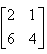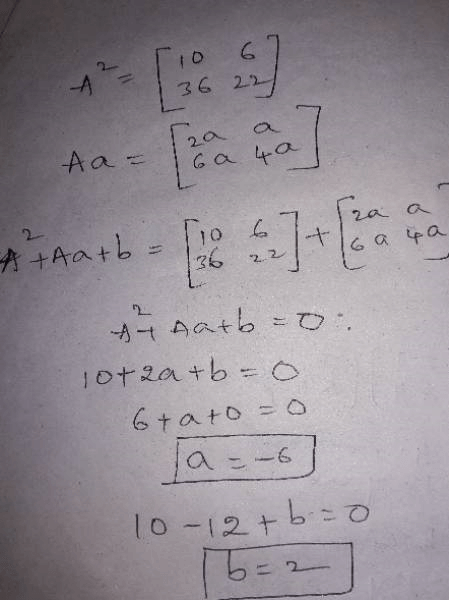Courses

# Matrix.. Class 12 Notes | EduRev

Created by: Shivani

## Class 12 : Matrix.. Class 12 Notes | EduRev

The document Matrix.. Class 12 Notes | EduRev is a part of Class 12 category.
All you need of Class 12 at this link: Class 12

If matrix A =and A2 + aA + b = O, then the values of a and b are:
• a)
a = – 6, b = 2
• b)
a = 6, b = – 2
• c)
a = 6, b = 2
• d)
a = – 6, b = – 2

Ref: https://edurev.in/question/459966/If-matrix-A-and-A2-aA-b-O-then-the-values-of-a-anhere frst we hv to calculate A^2...then add Aa and b to it...then equal to zero...u ll get the ans..
Offer running on EduRev: Apply code STAYHOME200 to get INR 200 off on our premium plan EduRev Infinity!

,

,

,

,

,

,

,

,

,

,

,

,

,

,

,

,

,

,

,

,

,

;